# Programming Languages and Compilers CS 421 Sasa Misailovic

• Slides: 76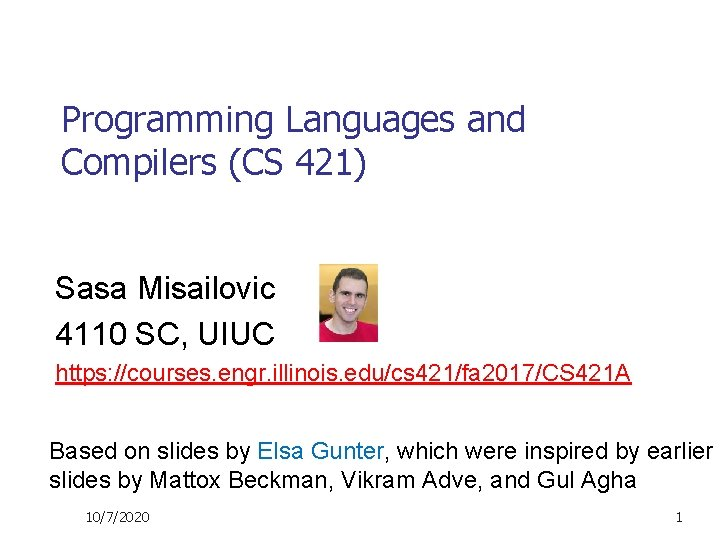Programming Languages and Compilers (CS 421) Sasa Misailovic 4110 SC, UIUC https: //courses. engr. illinois. edu/cs 421/fa 2017/CS 421 A Based on slides by Elsa Gunter, which were inspired by earlier slides by Mattox Beckman, Vikram Adve, and Gul Agha 10/7/2020 1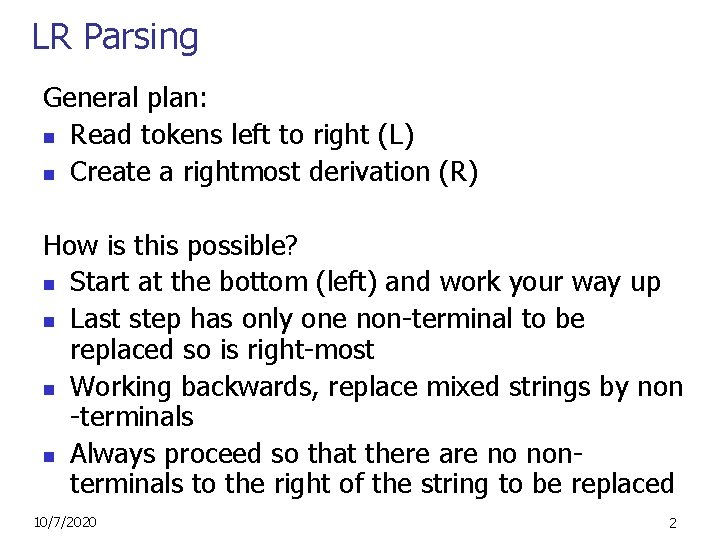LR Parsing General plan: n Read tokens left to right (L) n Create a rightmost derivation (R) How is this possible? n Start at the bottom (left) and work your way up n Last step has only one non-terminal to be replaced so is right-most n Working backwards, replace mixed strings by non -terminals n Always proceed so that there are no nonterminals to the right of the string to be replaced 10/7/2020 2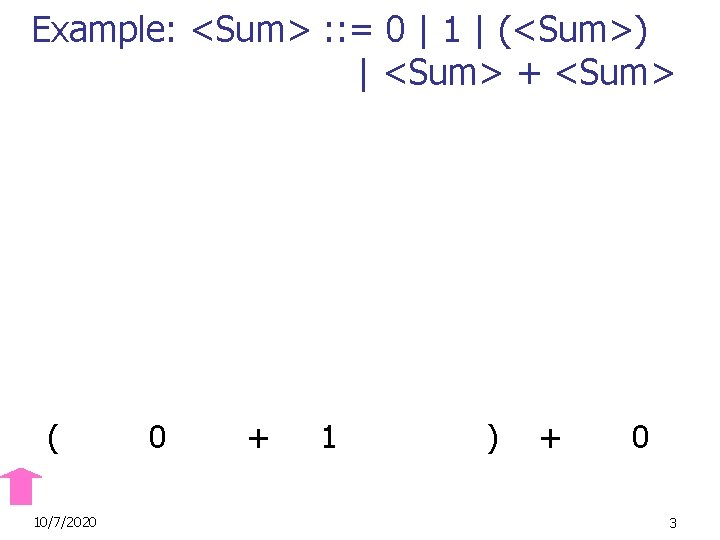Example: <Sum> : : = 0 | 1 | (<Sum>) | <Sum> + <Sum> ( 10/7/2020 0 + 1 ) + 0 3Example: <Sum> : : = 0 | 1 | (<Sum>) | <Sum> + <Sum> ( 10/7/2020 0 + 1 ) + 0 4Example: <Sum> : : = 0 | 1 | (<Sum>) | <Sum> + <Sum> ( 10/7/2020 0 + 1 ) + 0 5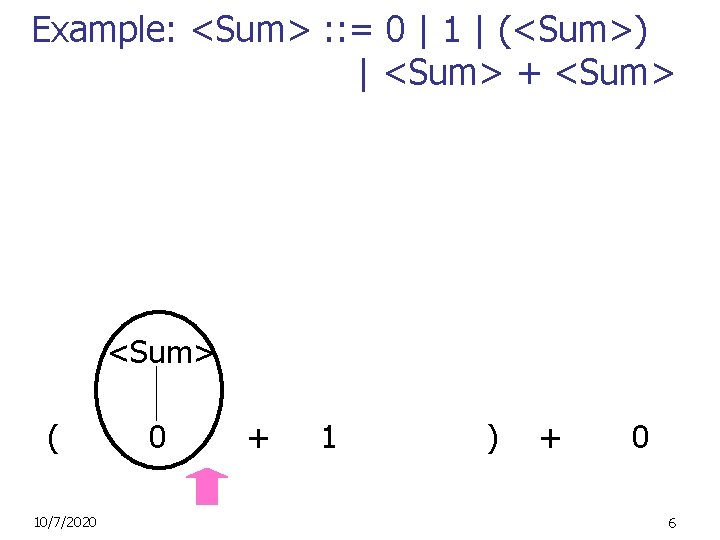Example: <Sum> : : = 0 | 1 | (<Sum>) | <Sum> + <Sum> ( 10/7/2020 0 + 1 ) + 0 6Example: <Sum> : : = 0 | 1 | (<Sum>) | <Sum> + <Sum> ( 10/7/2020 0 + 1 ) + 0 7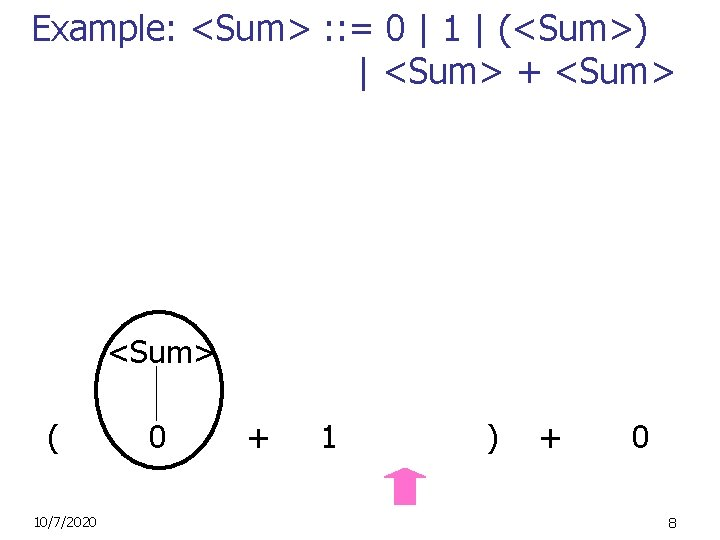Example: <Sum> : : = 0 | 1 | (<Sum>) | <Sum> + <Sum> ( 10/7/2020 0 + 1 ) + 0 8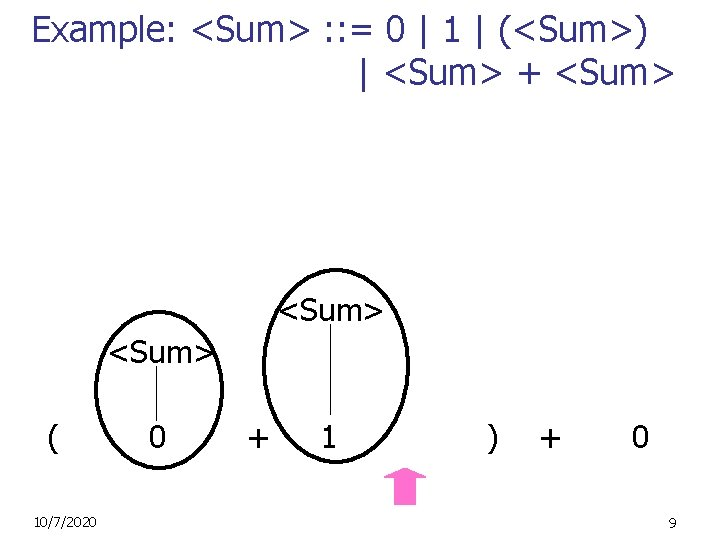Example: <Sum> : : = 0 | 1 | (<Sum>) | <Sum> + <Sum> ( 10/7/2020 0 + 1 ) + 0 9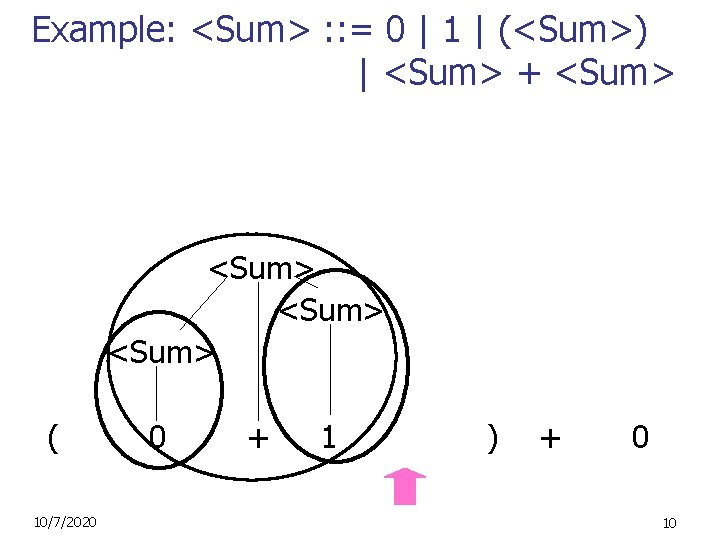Example: <Sum> : : = 0 | 1 | (<Sum>) | <Sum> + <Sum> ( 10/7/2020 0 + 1 ) + 0 10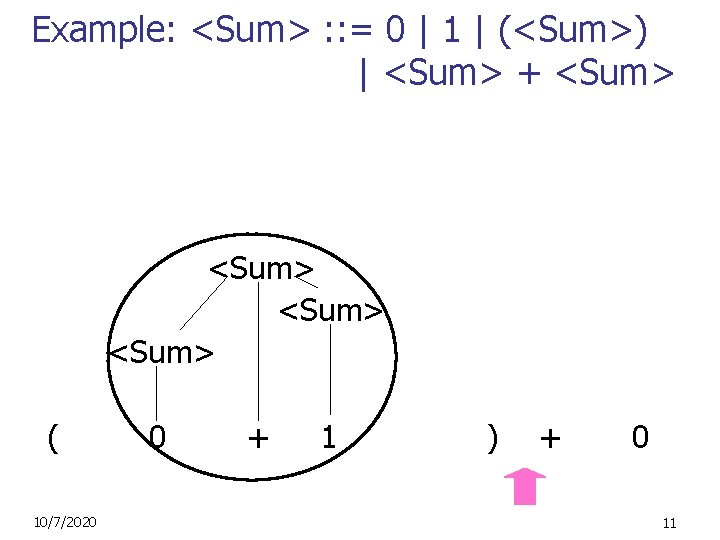Example: <Sum> : : = 0 | 1 | (<Sum>) | <Sum> + <Sum> ( 10/7/2020 0 + 1 ) + 0 11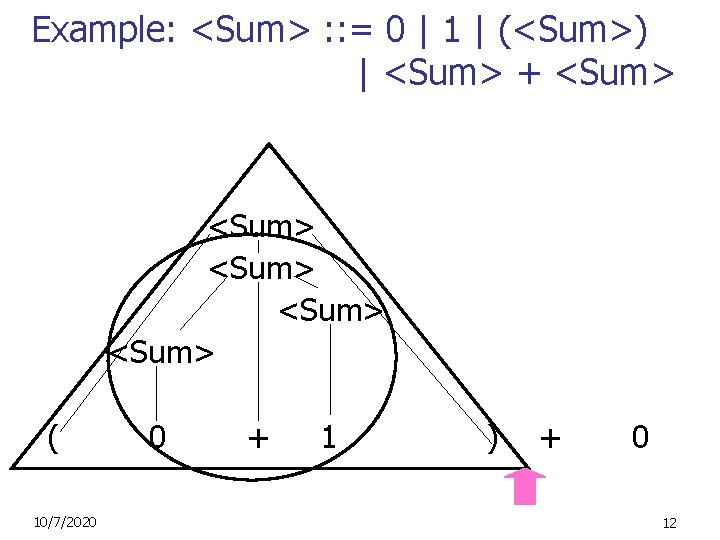Example: <Sum> : : = 0 | 1 | (<Sum>) | <Sum> + <Sum> <Sum> ( 10/7/2020 0 + 1 ) + 0 12Example: <Sum> : : = 0 | 1 | (<Sum>) | <Sum> + <Sum> <Sum> ( 10/7/2020 0 + 1 ) + 0 13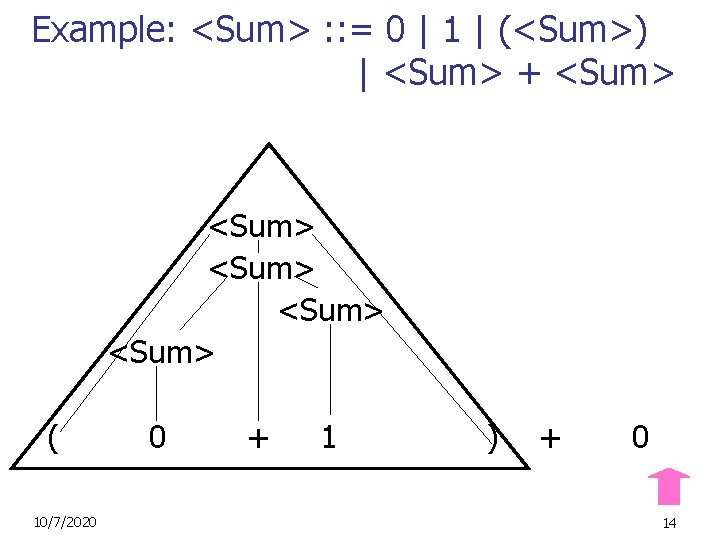Example: <Sum> : : = 0 | 1 | (<Sum>) | <Sum> + <Sum> <Sum> ( 10/7/2020 0 + 1 ) + 0 14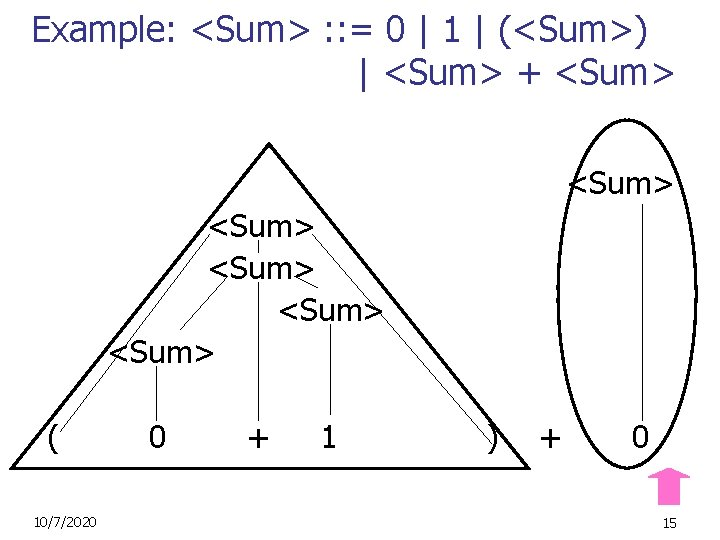Example: <Sum> : : = 0 | 1 | (<Sum>) | <Sum> + <Sum> <Sum> ( 10/7/2020 0 + 1 ) + 0 15Example: <Sum> : : = 0 | 1 | (<Sum>) | <Sum> + <Sum> <Sum> ( 10/7/2020 0 + 1 ) + 0 16Example: <Sum> : : = 0 | 1 | (<Sum>) | <Sum> + <Sum> <Sum> ( 10/7/2020 0 + 1 ) + 0 17LR Parsing Tables n Build a pair of tables, Action and Goto, from the grammar n This is the hardest part, we omit here (Also helpful to know pushdown automata) n n Rows labeled by states For Action, columns labeled by terminals and “end-of-tokens” marker n n (more generally strings of terminals of fixed length) For Goto, columns labeled by non-terminals 10/7/2020 18Action and Goto Tables n n Given a state and the next input, Action table says either n shift and go to state n, or n reduce by production k (explained in a bit) n accept or error Given a state and a non-terminal, Goto table says n go to state m 10/7/2020 19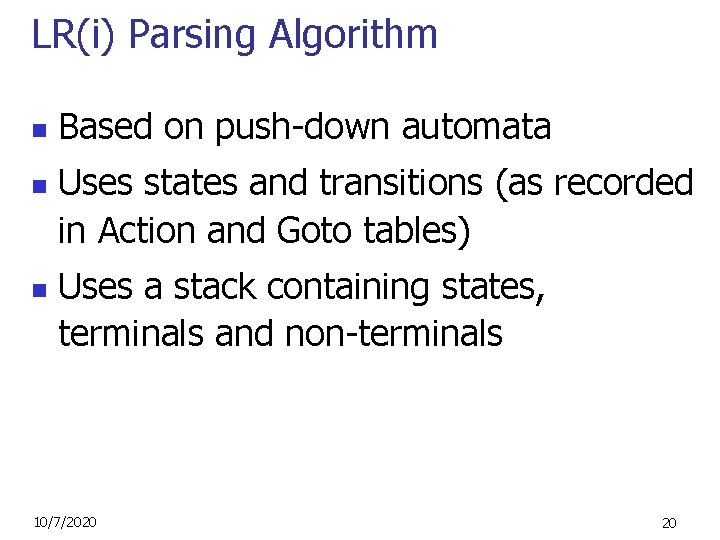LR(i) Parsing Algorithm n n n Based on push-down automata Uses states and transitions (as recorded in Action and Goto tables) Uses a stack containing states, terminals and non-terminals 10/7/2020 20LR(i) Parsing Algorithm 0. Insure token stream ends in special “endof-tokens” symbol 1. Start in state 1 with an empty stack 2. Push state(1) onto stack 3. Look at next i tokens from token stream (toks) (don’t remove yet) 4. If top symbol on stack is state(n), look up action in Action table at (n, toks) 10/7/2020 21LR(i) Parsing Algorithm 5. If action = shift m, a) Remove the top token from token stream and push it onto the stack b) Push state(m) onto stack c) Go to step 3 10/7/2020 22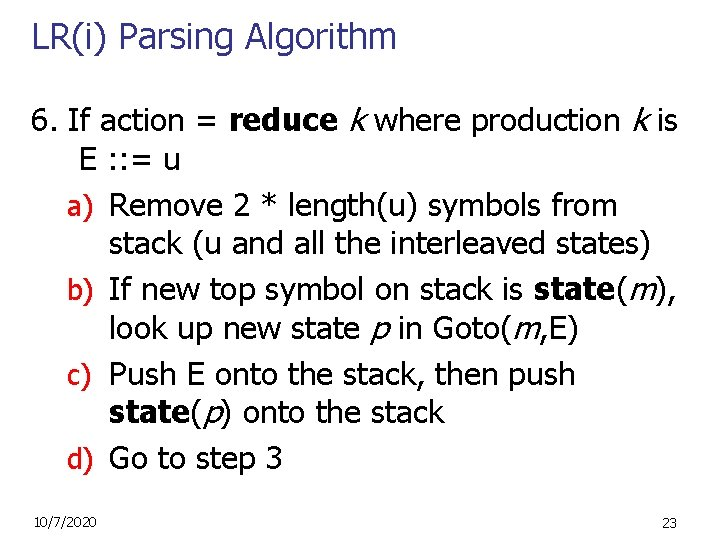LR(i) Parsing Algorithm 6. If action = reduce k where production k is E : : = u a) Remove 2 * length(u) symbols from stack (u and all the interleaved states) b) If new top symbol on stack is state(m), look up new state p in Goto(m, E) c) Push E onto the stack, then push state(p) onto the stack d) Go to step 3 10/7/2020 23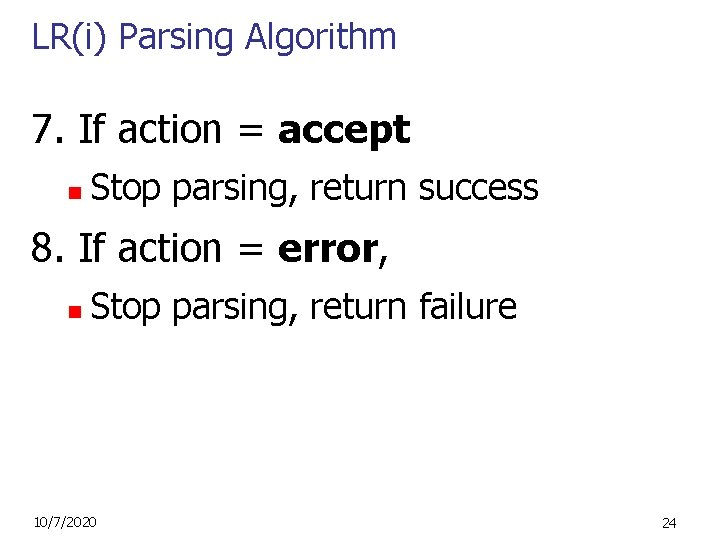LR(i) Parsing Algorithm 7. If action = accept n Stop parsing, return success 8. If action = error, n Stop parsing, return failure 10/7/2020 24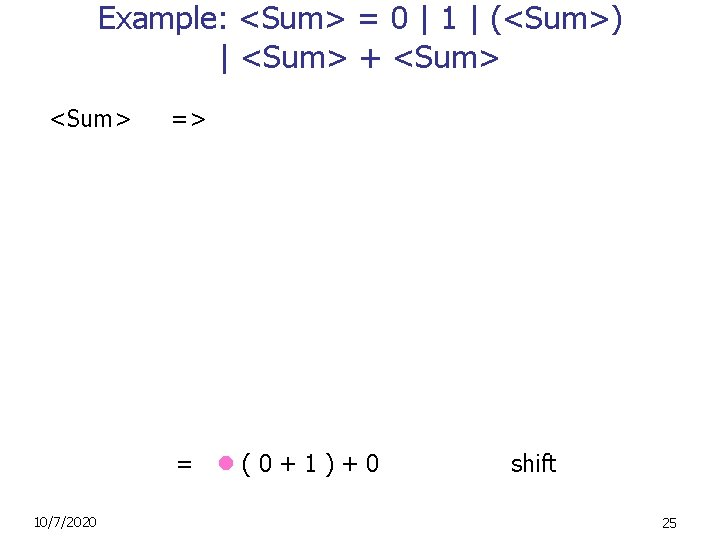Example: <Sum> = 0 | 1 | (<Sum>) | <Sum> + <Sum> => = (0+1)+0 10/7/2020 shift 25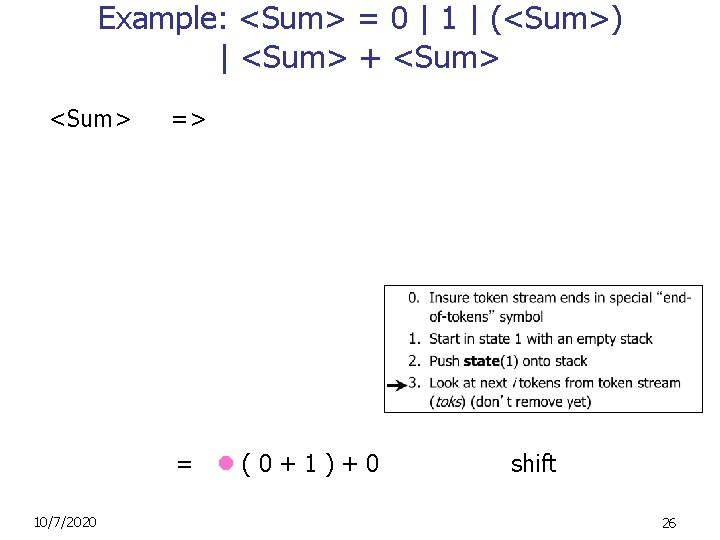Example: <Sum> = 0 | 1 | (<Sum>) | <Sum> + <Sum> => = (0+1)+0 10/7/2020 shift 26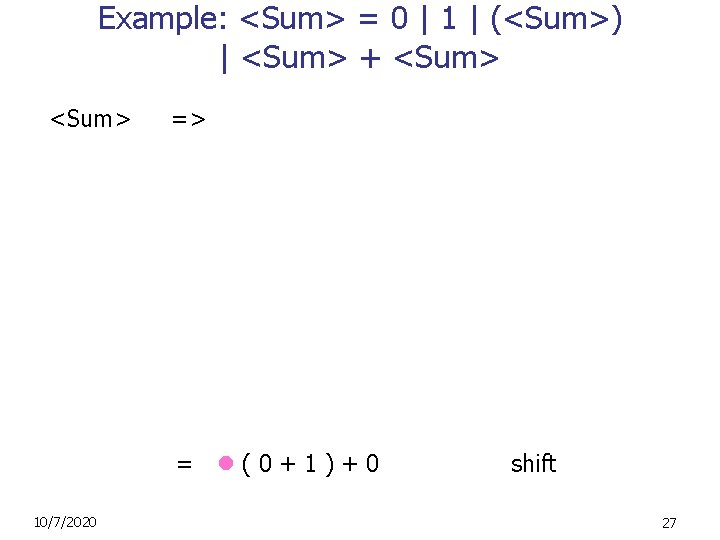Example: <Sum> = 0 | 1 | (<Sum>) | <Sum> + <Sum> => = (0+1)+0 10/7/2020 shift 27Example: <Sum> = 0 | 1 | (<Sum>) | <Sum> + <Sum> => = (0+1)+0 10/7/2020 shift 28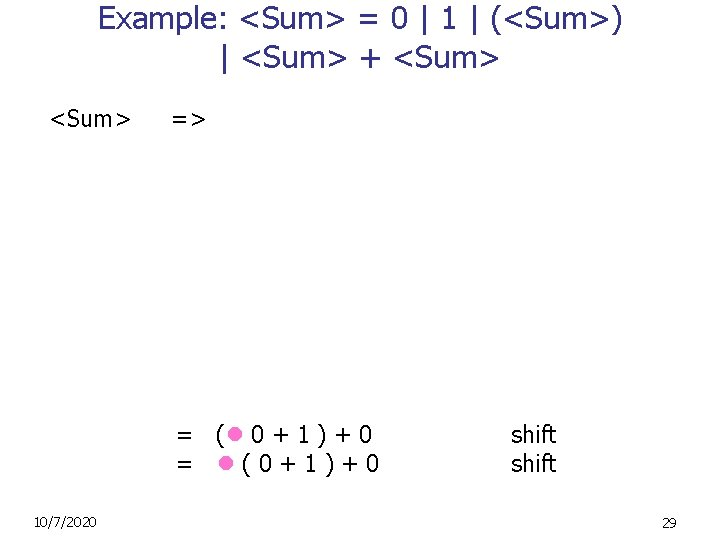Example: <Sum> = 0 | 1 | (<Sum>) | <Sum> + <Sum> => = ( 0 + 1 ) + 0 = (0+1)+0 10/7/2020 shift 29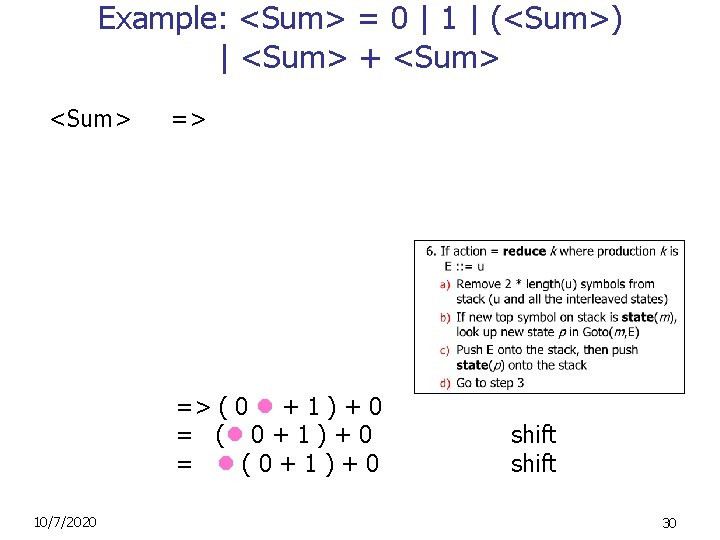Example: <Sum> = 0 | 1 | (<Sum>) | <Sum> + <Sum> => => ( 0 + 1 ) + 0 = ( 0 + 1 ) + 0 = (0+1)+0 10/7/2020 shift 30Example: <Sum> = 0 | 1 | (<Sum>) | <Sum> + <Sum> => => ( 0 + 1 ) + 0 = ( 0 + 1 ) + 0 = (0+1)+0 10/7/2020 reduce shift 31Example: <Sum> = 0 | 1 | (<Sum>) | <Sum> + <Sum> => = ( <Sum> + 1 ) + 0 => ( 0 + 1 ) + 0 = ( 0 + 1 ) + 0 = (0+1)+0 10/7/2020 reduce shift 32Example: <Sum> = 0 | 1 | (<Sum>) | <Sum> + <Sum> => = ( <Sum> + 1 ) + 0 => ( 0 + 1 ) + 0 = ( 0 + 1 ) + 0 = (0+1)+0 10/7/2020 shift reduce shift 33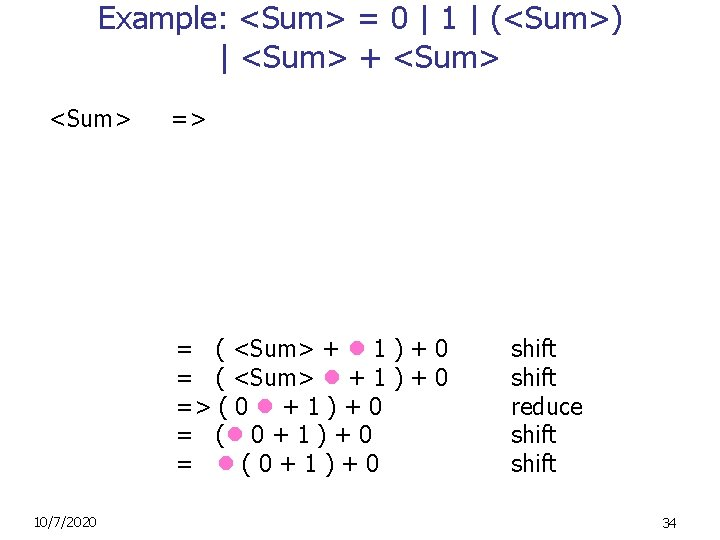Example: <Sum> = 0 | 1 | (<Sum>) | <Sum> + <Sum> => = ( <Sum> + 1 ) + 0 = ( <Sum> + 1 ) + 0 => ( 0 + 1 ) + 0 = ( 0 + 1 ) + 0 = (0+1)+0 10/7/2020 shift reduce shift 34Example: <Sum> = 0 | 1 | (<Sum>) | <Sum> + <Sum> => => ( <Sum> + 1 ) + 0 = ( <Sum> + 1 ) + 0 = ( <Sum> + 1 ) + 0 => ( 0 + 1 ) + 0 = ( 0 + 1 ) + 0 = (0+1)+0 10/7/2020 shift reduce shift 35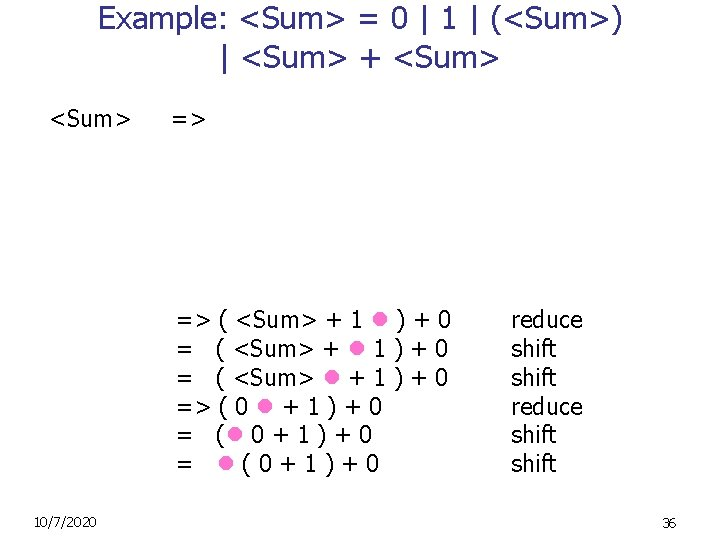Example: <Sum> = 0 | 1 | (<Sum>) | <Sum> + <Sum> => => ( <Sum> + 1 ) + 0 = ( <Sum> + 1 ) + 0 = ( <Sum> + 1 ) + 0 => ( 0 + 1 ) + 0 = ( 0 + 1 ) + 0 = (0+1)+0 10/7/2020 reduce shift 36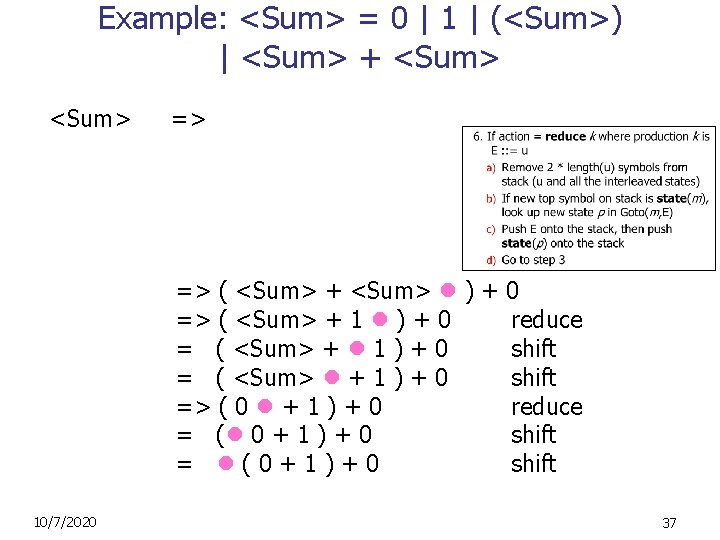Example: <Sum> = 0 | 1 | (<Sum>) | <Sum> + <Sum> => => ( <Sum> + <Sum> ) + 0 => ( <Sum> + 1 ) + 0 reduce = ( <Sum> + 1 ) + 0 shift = ( <Sum> + 1 ) + 0 shift => ( 0 + 1 ) + 0 reduce = ( 0 + 1 ) + 0 shift = (0+1)+0 shift 10/7/2020 37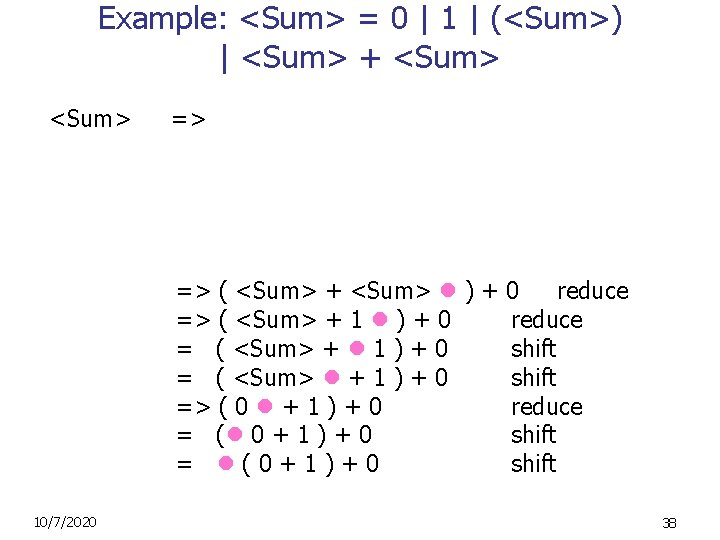Example: <Sum> = 0 | 1 | (<Sum>) | <Sum> + <Sum> => => ( <Sum> + <Sum> ) + 0 reduce => ( <Sum> + 1 ) + 0 reduce = ( <Sum> + 1 ) + 0 shift = ( <Sum> + 1 ) + 0 shift => ( 0 + 1 ) + 0 reduce = ( 0 + 1 ) + 0 shift = (0+1)+0 shift 10/7/2020 38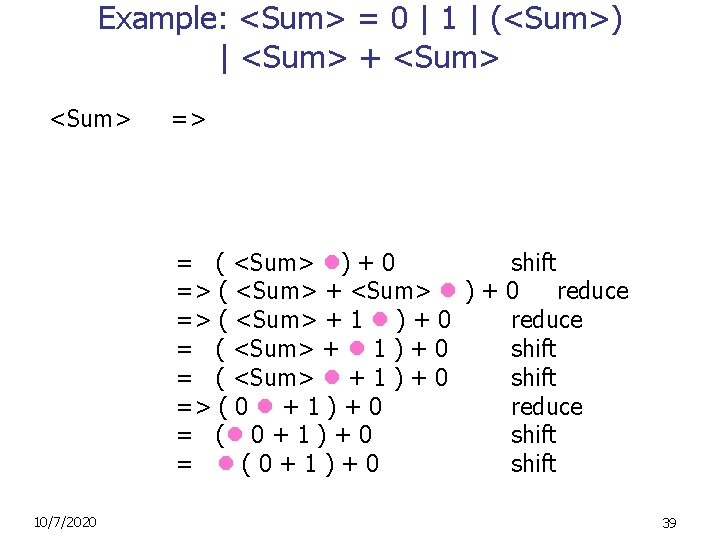Example: <Sum> = 0 | 1 | (<Sum>) | <Sum> + <Sum> => = ( <Sum> ) + 0 shift => ( <Sum> + <Sum> ) + 0 reduce => ( <Sum> + 1 ) + 0 reduce = ( <Sum> + 1 ) + 0 shift = ( <Sum> + 1 ) + 0 shift => ( 0 + 1 ) + 0 reduce = ( 0 + 1 ) + 0 shift = (0+1)+0 shift 10/7/2020 39Example: <Sum> = 0 | 1 | (<Sum>) | <Sum> + <Sum> => => ( <Sum> ) + 0 reduce = ( <Sum> ) + 0 shift => ( <Sum> + <Sum> ) + 0 reduce => ( <Sum> + 1 ) + 0 reduce = ( <Sum> + 1 ) + 0 shift = ( <Sum> + 1 ) + 0 shift => ( 0 + 1 ) + 0 reduce = ( 0 + 1 ) + 0 shift = (0+1)+0 shift 10/7/2020 40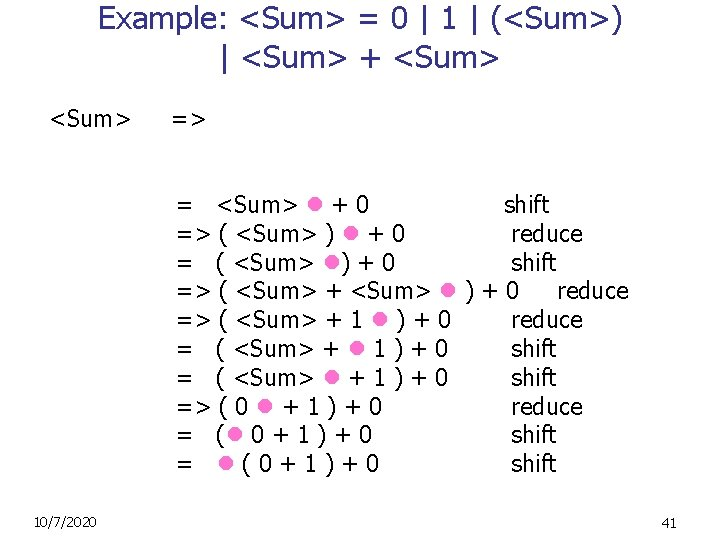Example: <Sum> = 0 | 1 | (<Sum>) | <Sum> + <Sum> => = <Sum> + 0 shift => ( <Sum> ) + 0 reduce = ( <Sum> ) + 0 shift => ( <Sum> + <Sum> ) + 0 reduce => ( <Sum> + 1 ) + 0 reduce = ( <Sum> + 1 ) + 0 shift = ( <Sum> + 1 ) + 0 shift => ( 0 + 1 ) + 0 reduce = ( 0 + 1 ) + 0 shift = (0+1)+0 shift 10/7/2020 41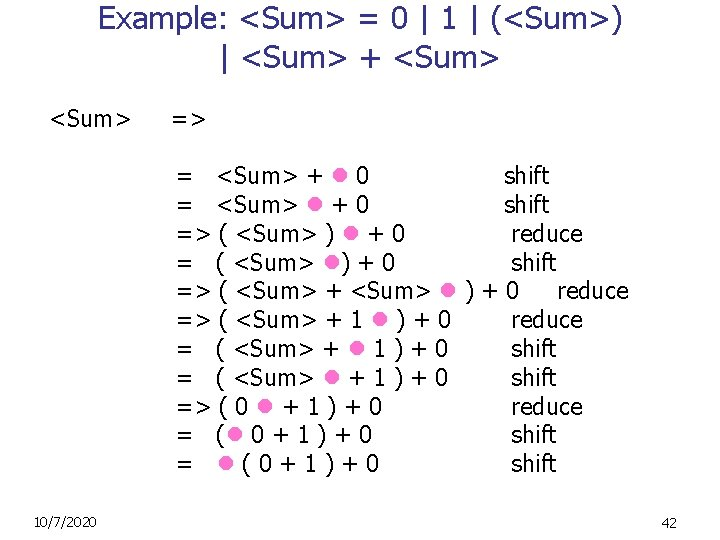Example: <Sum> = 0 | 1 | (<Sum>) | <Sum> + <Sum> => = <Sum> + 0 shift = <Sum> + 0 shift => ( <Sum> ) + 0 reduce = ( <Sum> ) + 0 shift => ( <Sum> + <Sum> ) + 0 reduce => ( <Sum> + 1 ) + 0 reduce = ( <Sum> + 1 ) + 0 shift = ( <Sum> + 1 ) + 0 shift => ( 0 + 1 ) + 0 reduce = ( 0 + 1 ) + 0 shift = (0+1)+0 shift 10/7/2020 42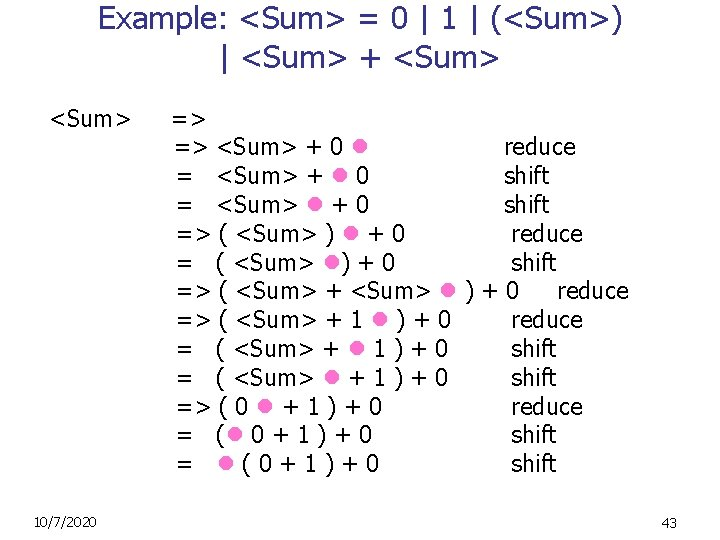Example: <Sum> = 0 | 1 | (<Sum>) | <Sum> + <Sum> => => <Sum> + 0 reduce = <Sum> + 0 shift = <Sum> + 0 shift => ( <Sum> ) + 0 reduce = ( <Sum> ) + 0 shift => ( <Sum> + <Sum> ) + 0 reduce => ( <Sum> + 1 ) + 0 reduce = ( <Sum> + 1 ) + 0 shift = ( <Sum> + 1 ) + 0 shift => ( 0 + 1 ) + 0 reduce = ( 0 + 1 ) + 0 shift = (0+1)+0 shift 10/7/2020 43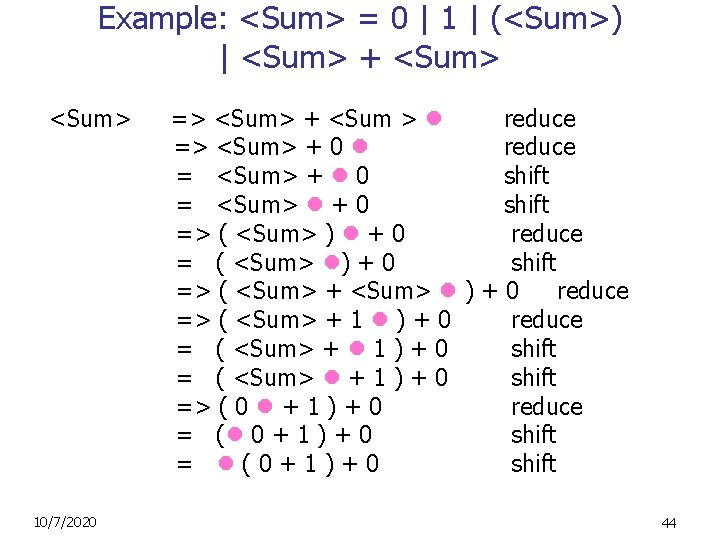Example: <Sum> = 0 | 1 | (<Sum>) | <Sum> + <Sum> => <Sum> + <Sum > reduce => <Sum> + 0 reduce = <Sum> + 0 shift = <Sum> + 0 shift => ( <Sum> ) + 0 reduce = ( <Sum> ) + 0 shift => ( <Sum> + <Sum> ) + 0 reduce => ( <Sum> + 1 ) + 0 reduce = ( <Sum> + 1 ) + 0 shift = ( <Sum> + 1 ) + 0 shift => ( 0 + 1 ) + 0 reduce = ( 0 + 1 ) + 0 shift = (0+1)+0 shift 10/7/2020 44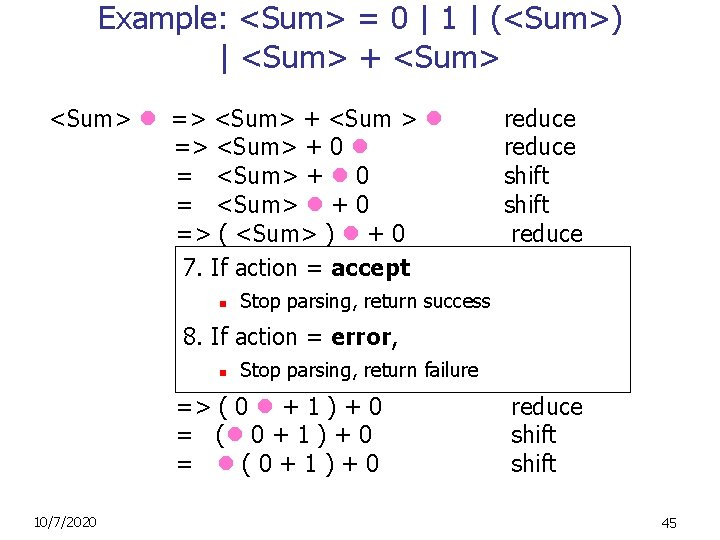Example: <Sum> = 0 | 1 | (<Sum>) | <Sum> + <Sum> => <Sum> + <Sum > reduce => <Sum> + 0 reduce = <Sum> + 0 shift = <Sum> + 0 shift => ( <Sum> ) + 0 reduce =7. If( <Sum> +0 shift action = ) accept => n( <Sum> + <Sum> )+0 reduce Stop parsing, return success => ( <Sum> + 1 ) + 0 reduce 8. If action = error, = ( <Sum> + 1 ) + 0 shift Stop parsing, = (n <Sum> +return 1 ) +failure 0 shift => ( 0 + 1 ) + 0 reduce = ( 0 + 1 ) + 0 shift = (0+1)+0 shift 10/7/2020 45Shift-Reduce Conflicts n n n Problem: can’t decide whether the action for a state and input character should be shift or reduce Caused by ambiguity in grammar Usually caused by lack of associativity or precedence information in grammar 10/7/2020 46Example: <Sum> = 0 | 1 | (<Sum>) | <Sum> + <Sum> -> <Sum> + <Sum> + 0 -> <Sum> + 1 + 0 reduce -> <Sum> + 1 + 0 shift -> <Sum> + 1 + 0 shift -> 0 + 1 + 0 reduce 0+1+0 shift 10/7/2020 47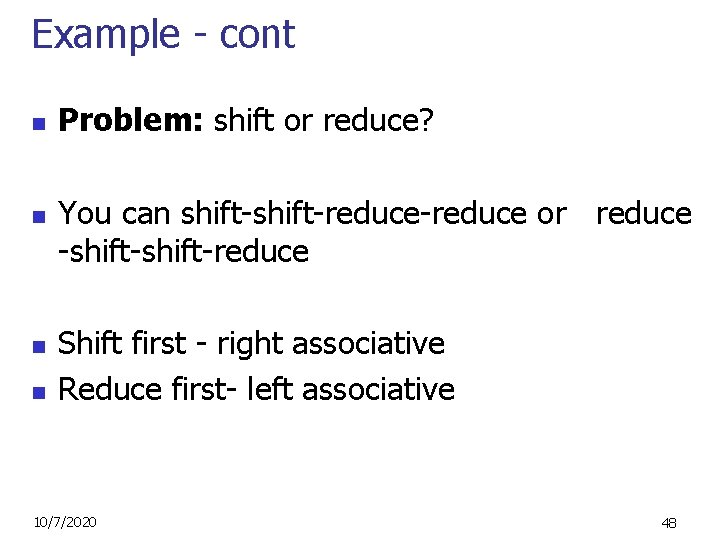Example - cont n n Problem: shift or reduce? You can shift-reduce-reduce or reduce -shift-reduce Shift first - right associative Reduce first- left associative 10/7/2020 48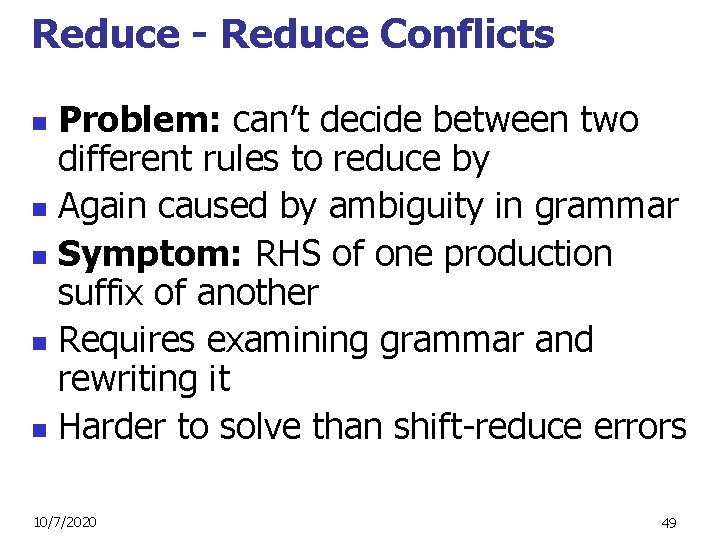Reduce - Reduce Conflicts Problem: can’t decide between two different rules to reduce by n Again caused by ambiguity in grammar n Symptom: RHS of one production suffix of another n Requires examining grammar and rewriting it n Harder to solve than shift-reduce errors n 10/7/2020 49Example n S : : = A | a. B abc ab c a bc abc n A : : = abc B : : = bc shift Problem: reduce by B : : = bc then by S : : = a. B, or by A: : = abc then S: : =A? 10/7/2020 50Ocamlyacc Output LR Parsers: • Suitable for arbitrary context-free grammars (and when the strings are in the language) • But: Debugging and customizing is a pain! 10/7/2020 51LL Parsing n n Recursive descent parsers are a class of parsers derived fairly directly from BNF grammars A recursive descent parser traces out a parse tree in top-down order, corresponding to a left-most derivation (LL - left-to-right scanning, leftmost derivation) 10/7/2020 52LL Parsing via Recursive Descent Parsers n n Each nonterminal in the grammar has a subprogram associated with it; the subprogram parses all phrases that the nonterminal can generate Each nonterminal in right-hand side of a rule corresponds to a recursive call to the associated subprogram 10/7/2020 53LL Parsing via Recursive Descent Parsers n n Each subprogram must be able to decide how to begin parsing by looking at the leftmost character in the string to be parsed n May do so directly, or indirectly by calling another parsing subprogram Recursive descent parsers, like other topdown parsers, cannot be built from leftrecursive grammars n n Sometimes can modify grammar to suit E. g. , from <sum> = <sum> + <term> to <sum> = <term> + sum 10/7/2020 54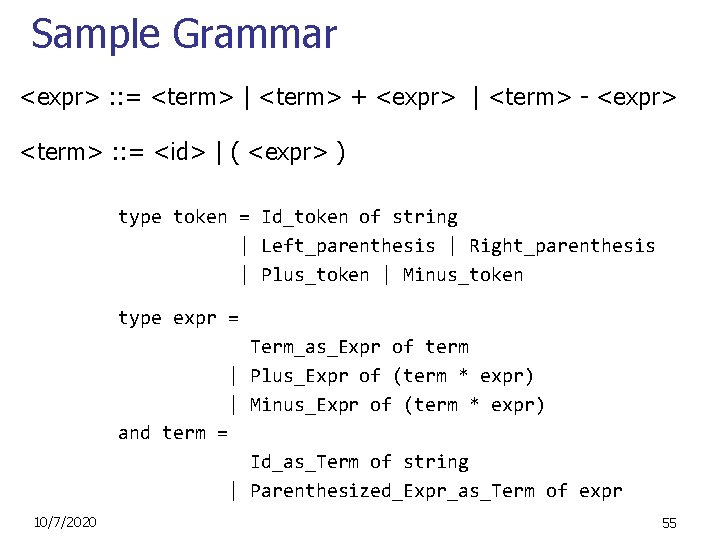Sample Grammar <expr> : : = <term> | <term> + <expr> | <term> - <expr> <term> : : = <id> | ( <expr> ) type token = Id_token of string | Left_parenthesis | Right_parenthesis | Plus_token | Minus_token type expr = Term_as_Expr of term | Plus_Expr of (term * expr) | Minus_Expr of (term * expr) and term = Id_as_Term of string | Parenthesized_Expr_as_Term of expr 10/7/2020 55Going Back to Sample Grammar <expr> : : = <term> | <term> + <expr> | <term> - <expr> <term> : : = <id> | ( <expr> ) In extended BNF notation : <expr> : : = <term> [(+ | -) <expr> ] <term> : : = <id> | ( <expr> ) Key observation: Parse tree of each rule has a unique leaf node n That way the parser knows which rule to immediately apply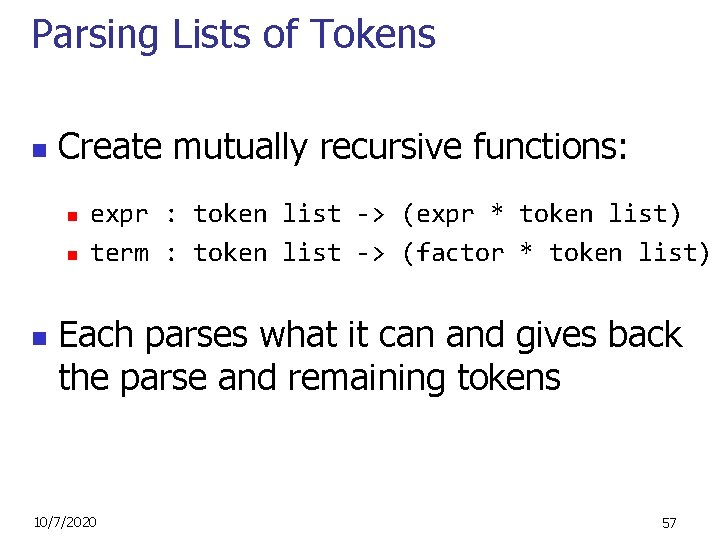Parsing Lists of Tokens n Create mutually recursive functions: n n n expr : token list -> (expr * token list) term : token list -> (factor * token list) Each parses what it can and gives back the parse and remaining tokens 10/7/2020 57Parsing Factor <term> : : = <id> | ( <expr> ) let rec term tokens = match tokens with (Id_token id_name) : : tokens_after_id -> ( Id_as_Factor id_name, tokens_after_id) | Left_parenthesis : : tokens -> (match expr tokens with (expr_parse, tokens_after_expr) -> (match tokens_after_expr with Right_parenthesis : : tokens_after_r -> (Parenthesized_Expr_as_Term expr_parse, tokens_after_r) )); ; 10/7/2020 58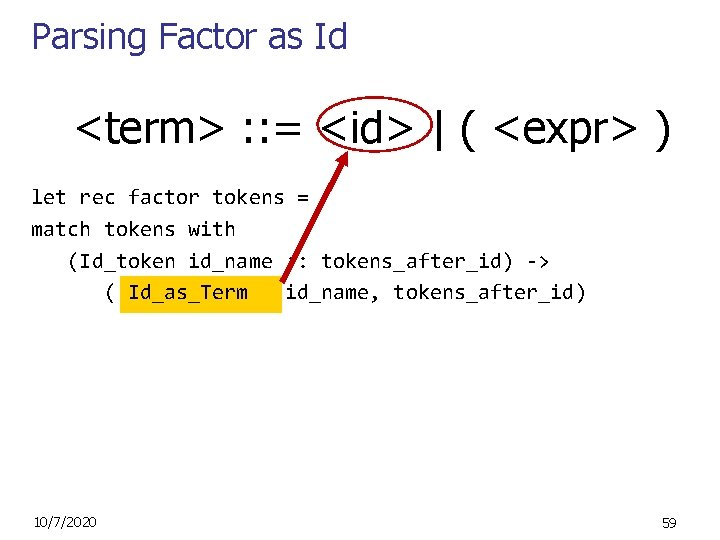Parsing Factor as Id <term> : : = <id> | ( <expr> ) let rec factor tokens = match tokens with (Id_token id_name : : tokens_after_id) -> ( Id_as_Term id_name, tokens_after_id) 10/7/2020 59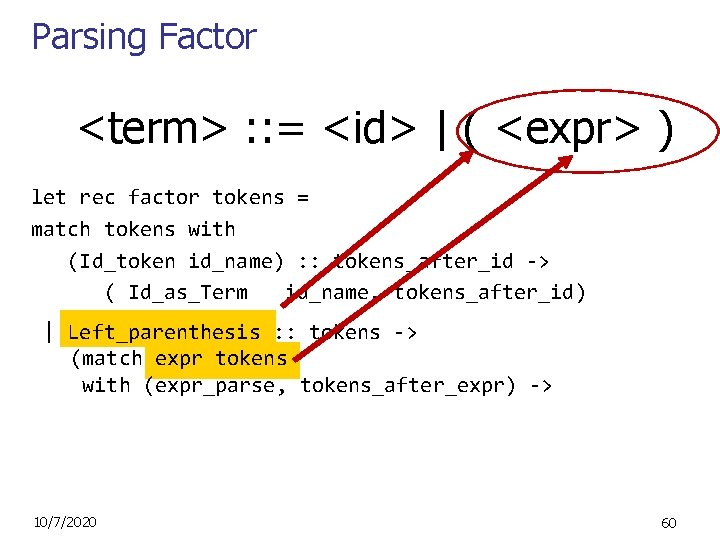Parsing Factor <term> : : = <id> | ( <expr> ) let rec factor tokens = match tokens with (Id_token id_name) : : tokens_after_id -> ( Id_as_Term id_name, tokens_after_id) | Left_parenthesis : : tokens -> (match expr tokens with (expr_parse, tokens_after_expr) -> 10/7/2020 60Parsing Factor <term> : : = <id> | ( <expr> ) let rec factor tokens = match tokens with (Id_token id_name) : : tokens_after_id -> ( Id_as_Term id_name, tokens_after_id) | Left_parenthesis : : tokens -> (match expr tokens with (expr_parse, tokens_after_expr) -> (match tokens_after_expr with Right_parenthesis : : tokens_after_r -> (Parenthesized_Expr_as_Term expr_parse, tokens_after_r) )) 10/7/2020 61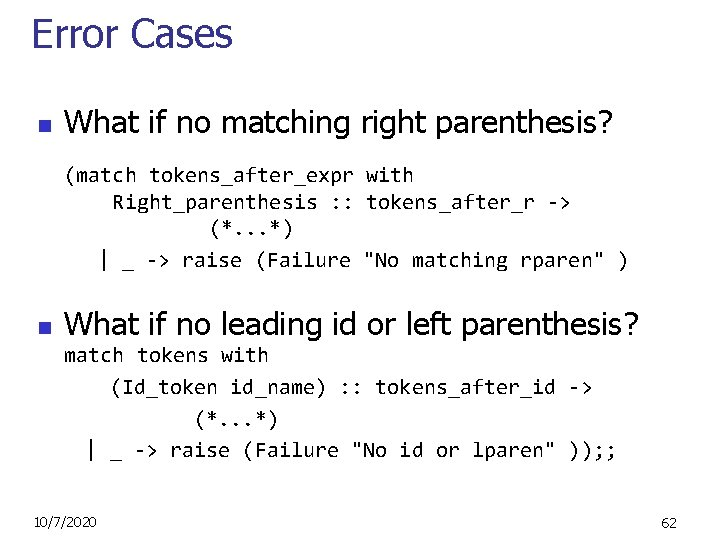Error Cases n What if no matching right parenthesis? (match tokens_after_expr with Right_parenthesis : : tokens_after_r -> (*. . . *) | _ -> raise (Failure "No matching rparen" ) n What if no leading id or left parenthesis? match tokens with (Id_token id_name) : : tokens_after_id -> (*. . . *) | _ -> raise (Failure "No id or lparen" )); ; 10/7/2020 62Parsing an Expression <expr> : : = <term> [( + | - ) <expr> ] and expr tokens = (match (term tokens) with (term_parse, tokens_after) -> (match tokens_after with Plus_token : : tokens_after_plus -> (*plus case*) | Minus_token : : tokens_after_minus -> (*minus case*) | _ -> (* this was either single term or error *) 10/7/2020 63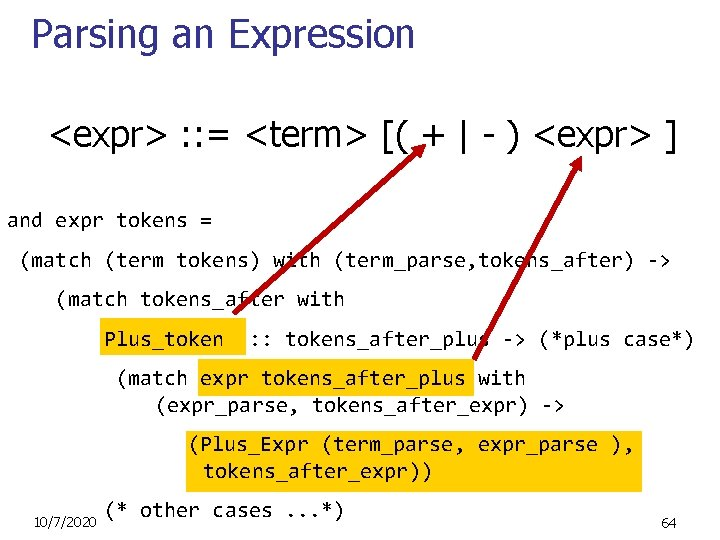Parsing an Expression <expr> : : = <term> [( + | - ) <expr> ] and expr tokens = (match (term tokens) with (term_parse, tokens_after) -> (match tokens_after with Plus_token : : tokens_after_plus -> (*plus case*) (match expr tokens_after_plus with (expr_parse, tokens_after_expr) -> (Plus_Expr (term_parse, expr_parse ), tokens_after_expr)) 10/7/2020 (* other cases. . . *) 64Parsing an Expression <expr> : : = <term> [( + | - ) <expr> ] and expr tokens = (match (term tokens) with (term_parse, tokens_after) -> (match tokens_after with Plus_token : : tokens_after_plus -> (*plus case*) | Minus_token : : tokens_after_minus -> (*minus case*) (match expr tokens_after_minus with ( expr_parse , tokens_after_expr) -> (Minus_Expr(term_parse, expr_parse), 10/7/2020 tokens_after_expr)) 65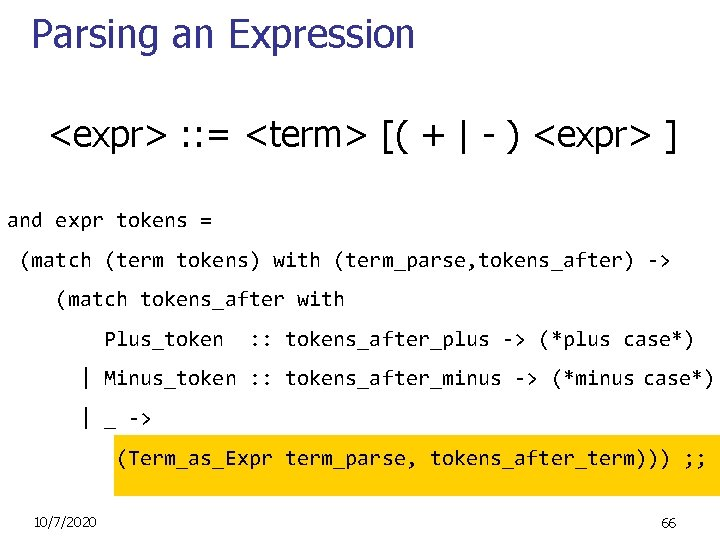Parsing an Expression <expr> : : = <term> [( + | - ) <expr> ] and expr tokens = (match (term tokens) with (term_parse, tokens_after) -> (match tokens_after with Plus_token : : tokens_after_plus -> (*plus case*) | Minus_token : : tokens_after_minus -> (*minus case*) | _ -> (Term_as_Expr term_parse, tokens_after_term))) ; ; 10/7/2020 66(a+b)+c-d expr [Left_parenthesis; Id_token "a”; Plus_token; Id_token "b”; Right_parenthesis; Plus_token; Id_token "c”; Minus_token; Id_token "d“ ]; ; 10/7/2020 67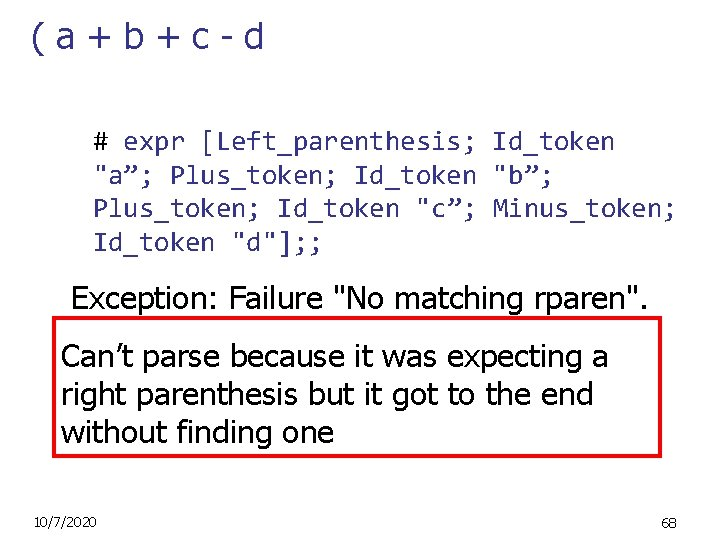(a+b+c-d # expr [Left_parenthesis; Id_token "a”; Plus_token; Id_token "b”; Plus_token; Id_token "c”; Minus_token; Id_token "d"]; ; Exception: Failure "No matching rparen". Can’t parse because it was expecting a right parenthesis but it got to the end without finding one 10/7/2020 68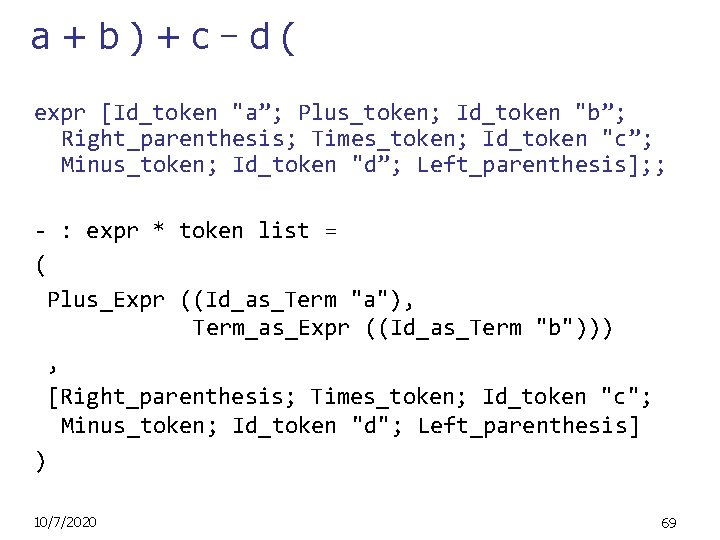a+b)+c–d( expr [Id_token "a”; Plus_token; Id_token "b”; Right_parenthesis; Times_token; Id_token "c”; Minus_token; Id_token "d”; Left_parenthesis]; ; - : expr * token list = ( Plus_Expr ((Id_as_Term "a"), Term_as_Expr ((Id_as_Term "b"))) , [Right_parenthesis; Times_token; Id_token "c"; Minus_token; Id_token "d"; Left_parenthesis] ) 10/7/2020 69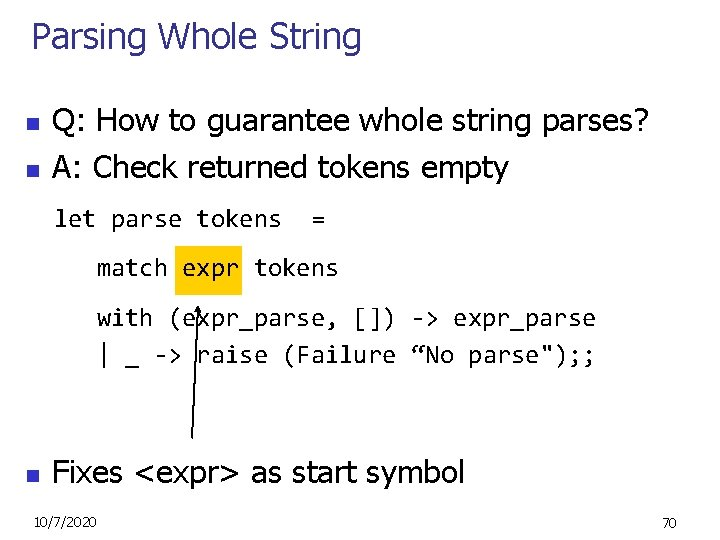Parsing Whole String n n Q: How to guarantee whole string parses? A: Check returned tokens empty let parse tokens = match expr tokens with (expr_parse, []) -> expr_parse | _ -> raise (Failure “No parse"); ; n Fixes <expr> as start symbol 10/7/2020 70Problems for Recursive-Descent Parsing n n Left Recursion: A : : = Aw translates to a subroutine that loops forever Indirect Left Recursion: A : : = Bw B : : = Av causes the same problem 10/7/2020 71Problems for Recursive-Descent Parsing n Parser must always be able to choose the next action based only on the very next token Pairwise Disjointedness Test: n Can we always determine which rule (in the non-extended BNF) to choose based on just the first token n n For each rule A : : = y Calculate FIRST (y) = {a | y =>* aw} { | if y =>* } For each pair of rules A : : = y and A : : = z, require FIRST(y) FIRST(z) = { } 72Example Grammar: <S> : : = <A> a <B> b <A> : : = <A> b | b <B> : : = a <B> | a FIRST (<A> b) = {b} FIRST (b) = {b} Rules for <A> not pairwise disjoint 10/7/2020 73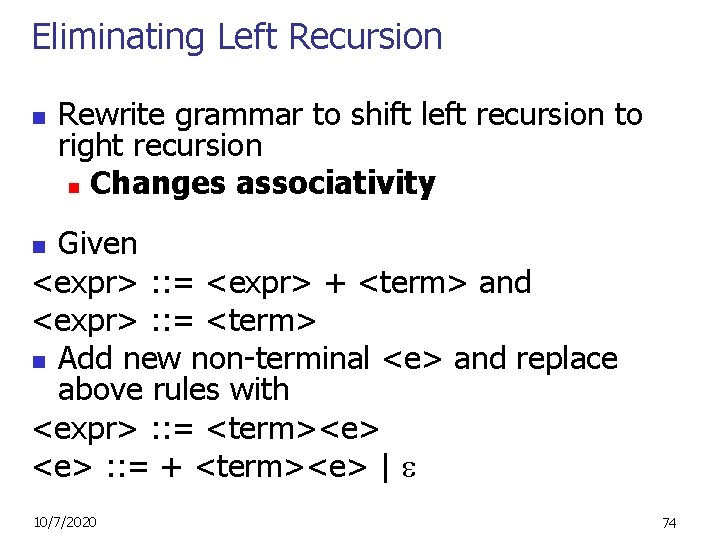Eliminating Left Recursion n Rewrite grammar to shift left recursion to right recursion n Changes associativity Given <expr> : : = <expr> + <term> and <expr> : : = <term> n Add new non-terminal <e> and replace above rules with <expr> : : = <term><e> : : = + <term><e> | n 10/7/2020 74Factoring Grammar n n n Test too strong: Can’t handle <expr> : : = <term> [ ( + | - ) <expr> ] Answer: Add new non-terminal and replace above rules by <expr> : : = <term><e> : : = + <term><e> : : = - <term><e> : : = You are delaying the decision point 10/7/2020 75Example Both <A> and <B> have problems: Transform grammar to: <S> : : = <A> a <B> b <A> : : = <A> b | b <A> : : -= b<A 1> : : b<A 1> | <B> : : = a <B> | a <B> : : = a<B 1> | 10/7/2020 76